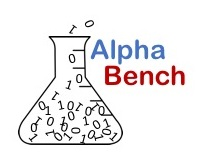## Using pandas for Stock Price Analysis

Video tutorial demonstrating data analysis and transformation using the Python programming language and pandas DataFrame. This is a follow-on video for using pandas rolling method for moving averages and rolling statistics.

You may also be interested in using Python to create a stock correlation matrix.

## Python pandas topics

• Calculate Moving Averages
• Calculate expected change
• Calculate magnitude of change (actual vs. expected)
• Create histogram
• Create scatter plot to visualize actual change vs. magnitude